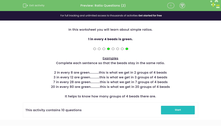# Ratio Questions (2)

In this worksheet, students learn about simple ratios.Key stage:  KS 2

Curriculum topic:   Ratio and Proportion

Curriculum subtopic:   Solve Ratio Problems

Popular topics:   Ratio worksheets

Difficulty level:#### Worksheet Overview

In this worksheet you will learn about simple ratios.

1 in every 4 beads is green.Examples

Complete each sentence so that the beads stay in the same ratio.

2 in every 8 are green...........this is what we get in 2 groups of 4 beads

3 in every 12 are green...........this is what we get in 3 groups of 4 beads

7 in every 28 are green...........this is what we get in 7 groups of 4 beads

20 in every 80 are green...........this is what we get in 20 groups of 4 beads

It helps to know how many groups of 4 beads there are.

Question:

If there are 12 green beads, how many beads are not green?

36

To work this question out, we can use multiplication facts:

The ratio is 3:1 (white:green)

If 12 beads are green, 1 x 12 = 12, so we do 3 x 12 = 36 to get the number of white beads.

### What is EdPlace?

We're your National Curriculum aligned online education content provider helping each child succeed in English, maths and science from year 1 to GCSE. With an EdPlace account you’ll be able to track and measure progress, helping each child achieve their best. We build confidence and attainment by personalising each child’s learning at a level that suits them.

Get started